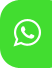## Product Detail## Ignou Solved Assignment For Che 04 Physical Chemistry

University  IGNOU
Service Type Solved Assignment
Course BA(Chemistry)
Semester
Short Name or Subject Code Che 04 Physical Chemistry
Product BA(Chemistry) of Solved Assignment (IGNOU)
Pattern
Price
Click to view price

Note: Answer all the questions given below. The marks are indicated in the brackets.

1. a) What is the value of density of mercury non-SI units? Convert and express this quantity in SI unit.

b) List the factors on which the amount of a gas adsorbed on a solid depends.

2. State Dalton’s law of partial pressure. Derive its mathematical form.

3. Derive equation of corresponding states.

4. What are liquid crystals? Explain their different types.

5. State Bragg law. List the assumptions made by Bragg in deriving Bragg equation.

6. Derive the equation for the work of expansion for an isothermal reversible process.

7. Define enthalpy of combustion. Calculate the enthalpy of combustion of methanol if the enthalpies of formation of CO2, H2O and CH3OH are, respectively, 393.5 kJ mol1 , 285.8 kJ mol1 and 239.0 kJ mol1 .

8. Draw and explain the steps involved in Carnot cycle.

9. Discuss the dependence of chemical potential on temperature and pressure.

10. What are the characteristics of ideal and non-ideal solutions? Give one example of each type of these solutions.

11. Give the thermodynamic derivation of the distribution law.

12. What is relative lowering of vapor pressure? How can lowering of vapour pressure be measured using static method?

13. Discuss the phase diagram of water.

14. State Le Chatelier’s principle. Discuss the effect of change of concentration on the following reaction: H2(g) + I2(g) ⇌ 2HI(g)

15. For the hydrolysis of a salt of a weak acid and a weak base, show that a b w K K K h K 

16. How can ionic product be determined using conductivity measurements? Explain.

17. Discuss the working of metal-insoluble salt electrode by taking a suitable example.

18. Derive integrated rate law for the first order reactions.

19. Briefly explain the laws of photochemistry.

20. Discuss the methods of preparation of lyophilic colloidal dispersions using dispersion methods.